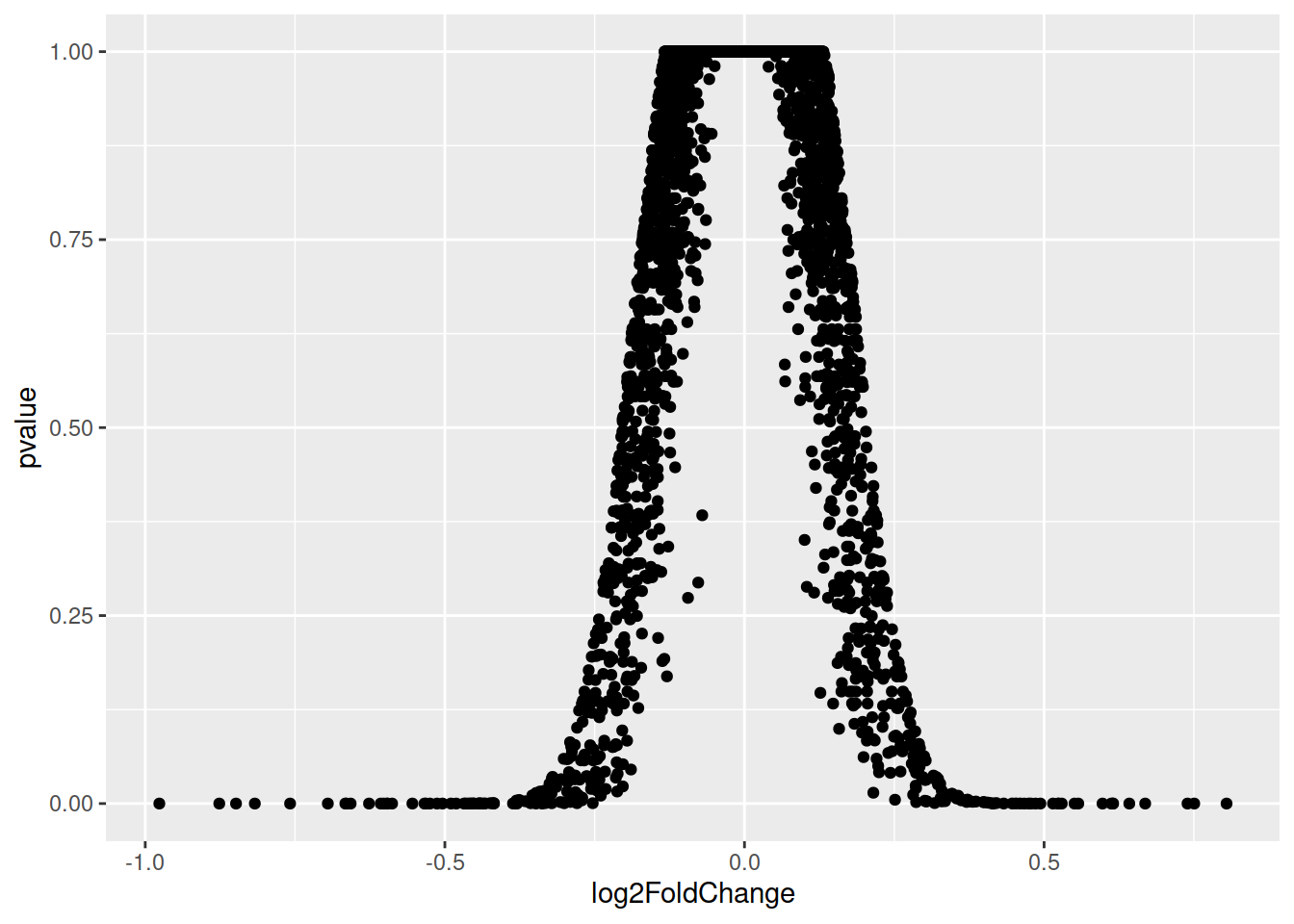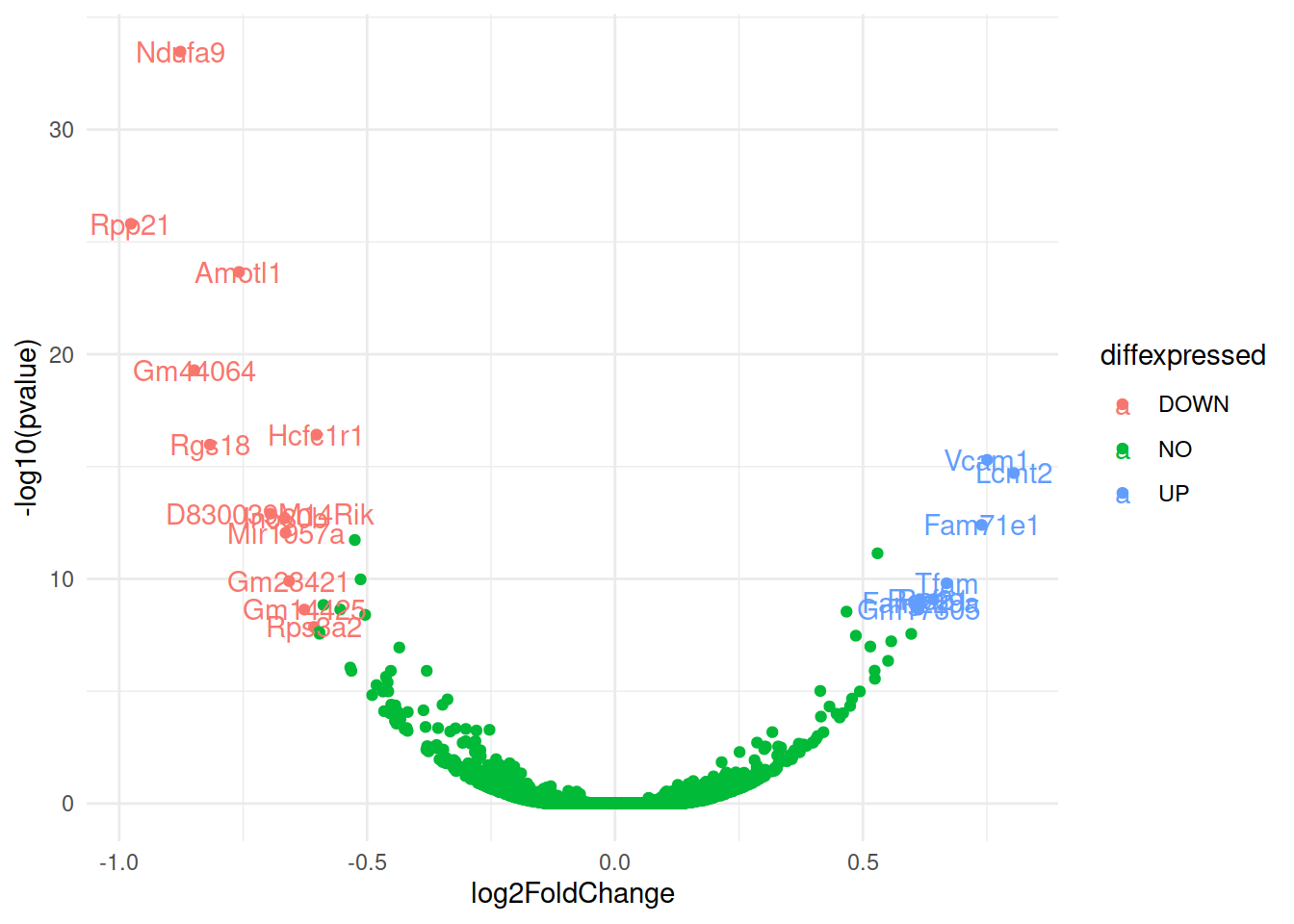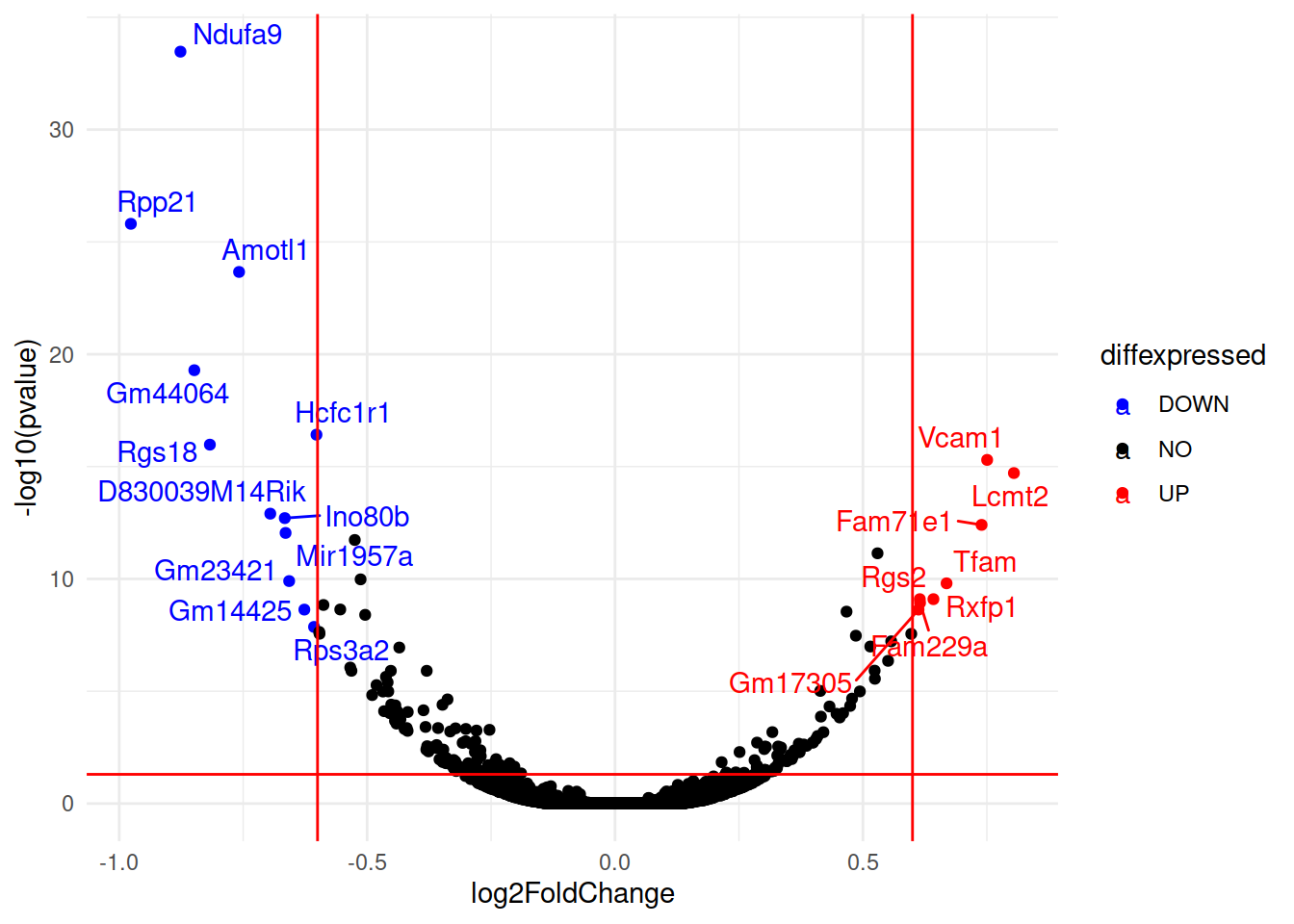## 16.10 demo: volcano plots

A volcano plot is a type of scatter plot represents differential expression of features (genes for example): on the x-axis we typically find the fold change and on the y-axis the p-value.

``````# Download the data we will use for plotting

# The RDS format is used to save a single R object to a file, and to restore it.
# Extract that object in the current session:

# remove rows that contain NA values
de <- tmp[complete.cases(tmp), ]``````
``````# The basic scatter plot: x is "log2FoldChange", y is "pvalue"
ggplot(data=de, aes(x=log2FoldChange, y=pvalue)) + geom_point()``````Doesn’t look quite like a Volcano plot…
Convert the p-value into a -log10(p-value)

``````# Convert directly in the aes()
p <- ggplot(data=de, aes(x=log2FoldChange, y=-log10(pvalue))) + geom_point()``````
``````# Add more simple "theme"
p <- ggplot(data=de, aes(x=log2FoldChange, y=-log10(pvalue))) + geom_point() + theme_minimal()``````
``````# Add vertical lines for log2FoldChange thresholds, and one horizontal line for the p-value threshold
p2 <- p + geom_vline(xintercept=c(-0.6, 0.6), col="red") +
geom_hline(yintercept=-log10(0.05), col="red")``````
``````# The significantly differentially expressed genes are the ones found in the upper-left and upper-right corners.
# Add a column to the data frame to specify if they are UP- or DOWN- regulated (log2FoldChange respectively positive or negative)

# add a column of NAs
de\$diffexpressed <- "NO"
# if log2Foldchange > 0.6 and pvalue < 0.05, set as "UP"
de\$diffexpressed[de\$log2FoldChange > 0.6 & de\$pvalue < 0.05] <- "UP"
# if log2Foldchange < -0.6 and pvalue < 0.05, set as "DOWN"
de\$diffexpressed[de\$log2FoldChange < -0.6 & de\$pvalue < 0.05] <- "DOWN"

# Re-plot but this time color the points with "diffexpressed"
p <- ggplot(data=de, aes(x=log2FoldChange, y=-log10(pvalue), col=diffexpressed)) + geom_point() + theme_minimal()

p2 <- p + geom_vline(xintercept=c(-0.6, 0.6), col="red") +
geom_hline(yintercept=-log10(0.05), col="red")``````
``````## Change point color

# 1. by default, it is assigned to the categories in an alphabetical order):
p3 <- p2 + scale_color_manual(values=c("blue", "black", "red"))

# 2. to automate a bit: ceate a named vector: the values are the colors to be used, the names are the categories they will be assigned to:
mycolors <- c("blue", "red", "black")
names(mycolors) <- c("DOWN", "UP", "NO")
p3 <- p2 + scale_colour_manual(values = mycolors)``````
``````# Now write down the name of genes beside the points...
# Create a new column "delabel" to de, that will contain the name of genes differentially expressed (NA in case they are not)
de\$delabel <- NA
de\$delabel[de\$diffexpressed != "NO"] <- de\$gene_symbol[de\$diffexpressed != "NO"]

ggplot(data=de, aes(x=log2FoldChange, y=-log10(pvalue), col=diffexpressed, label=delabel)) +
geom_point() +
theme_minimal() +
geom_text()````````````# Finally, we can organize the labels nicely using the "ggrepel" package and the geom_text_repel() function